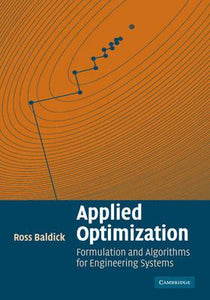# Applied Optimization : Formulation and Algorithms for Engineering Systems by Applied Optimization : Formulation and Algorithms for Engineering Systems

• R 900.00
Unit price per
Tax included.

ISBN: 9780521100281

CAMBRIDGE UNIVERSITY PRESS, 18 January 2009

Soft cover, 792 pages

The starting point in the formulation of any numerical problem is to take an intuitive idea about the problem in question and to translate it into precise mathematical language. This book provides step-by-step descriptions of how to formulate numerical problems and develops techniques for solving them. A number of engineering case studies motivate the development of efficient algorithms that involve, in some cases, transformation of the problem from its initial formulation into a more tractable form. Five general problem classes are considered: linear systems of equations, non-linear systems of equations, unconstrained optimization, equality-constrained optimization and inequality-constrained optimization. The book contains many worked examples and homework exercises and is suitable for students of engineering or operations research taking courses in optimization. Supplementary material including solutions, lecture slides and appendices are available online at www.cambridge.org/9780521855648.